## Sunday, February 15, 2015

### Perhaps the last (wishful thinking?) bug fix for reroot function for trees with node labels

I have just posted yet another bug fix for the phytools function `reroot` for trees containing node labels. This bug (reported by a helpful user) had the effect of causing one of the edge lengths descended from the new root to be wrong.

All are basically related to the same underlying issue which is that the ape function `drop.tip(...,trim.internal=FALSE)` will label the stem of a trimmed clade with the tip label `"NA"` when node labels are absent, but the node label itself when present. All of the internals used by `reroot` were originally built around the assumption that the trimmed edge would be labeled `"NA"`. Unfortunately, this created issues for multiple internals - which I have been peeling back & fixing one-by-one.

This update is also in the latest (non-CRAN) phytools version which, as always, can be obtained from the phytools webpage.

Here is a quick demo of the bug & its fix.

First `reroot` behaving properly for a tree without node labels:

``````library(phytools)
## simulate a tree
set.seed(1)
tree<-rtree(n=26,tip.label=LETTERS)
plotTree(tree)
nodelabels()
``````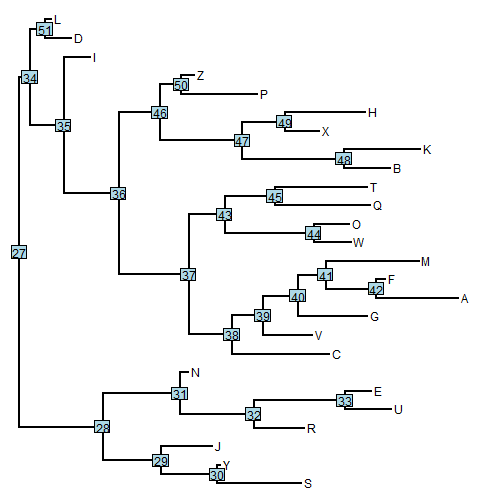``````## here with a tree without node labels
t1<-reroot(tree,31,0.5*tree\$edge.length[which(tree\$edge[,2]==31)])
plotTree(t1)
``````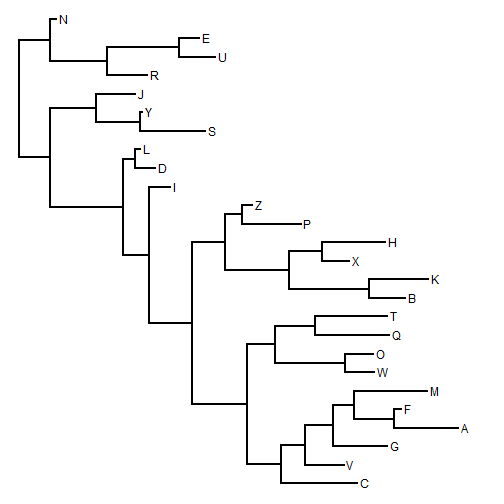``````## we re-rooting in the middle of an edge so
## these should be equal
t1\$edge.length[which(t1\$edge[,1]==(Ntip(t1)+1))]
``````
``````##  0.3661569 0.3661569
``````

OK, now here it is misbehaving. The tree is re-rooted in the right spot, but the edge lengths descended from the root are wrong:

``````## add node labels
tree\$node.label<-paste("n",1:tree\$Nnode+Ntip(tree),sep="")
plotTree(tree)
nodelabels(tree\$node.label)
``````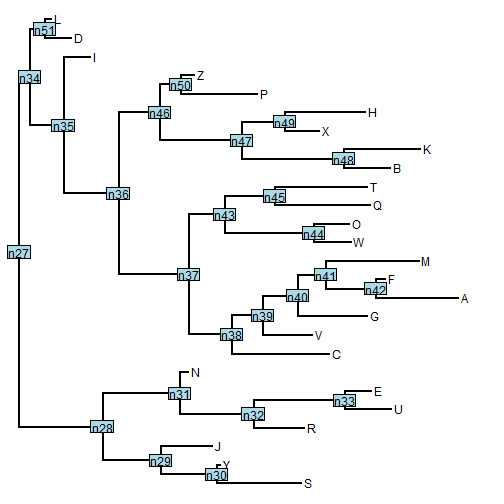``````t2<-reroot(tree,31,0.5*tree\$edge.length[which(tree\$edge[,2]==31)])
plotTree(t2) ## look at the edge lengths
nodelabels(t2\$node.label)
``````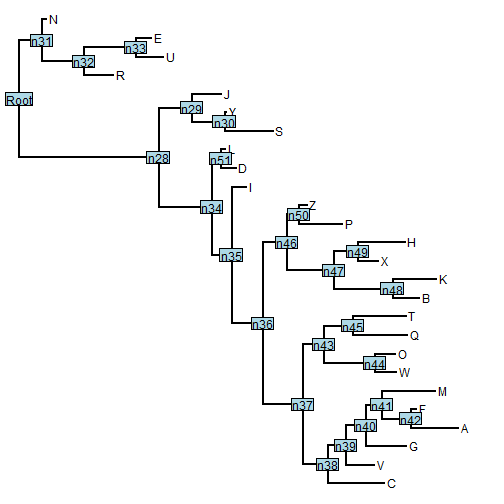``````## these should be equal, but they are not
t2\$edge.length[which(t1\$edge[,1]==(Ntip(t2)+1))]
``````
``````##  2.2862548 0.3661569
``````

OK, now let's load the fix and try again:

``````detach("package:phytools", unload=TRUE)
install.packages("phytools_0.4-49.tar.gz",type="source")
``````
``````## Installing package into 'C:/Users/Liam/Documents/R/win-library/3.1'
## (as 'lib' is unspecified)
## inferring 'repos = NULL' from 'pkgs'
``````
``````library(phytools)
t2<-reroot(tree,31,0.5*tree\$edge.length[which(tree\$edge[,2]==31)])
plotTree(t2)
nodelabels(t2\$node.label)
``````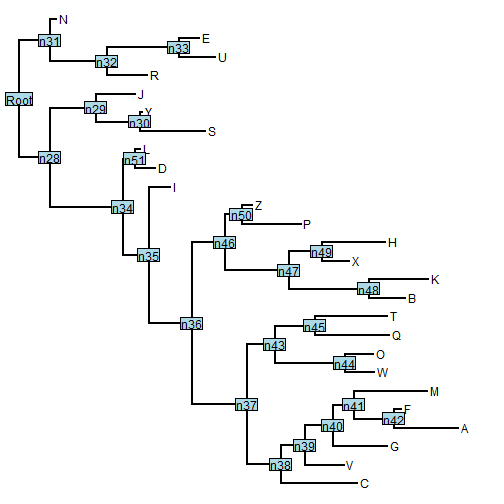``````## these should be equal
t2\$edge.length[which(t1\$edge[,1]==(Ntip(t2)+1))]
``````
``````##  0.3661569 0.3661569
``````

That's it.